# Verbal Reasoning - Character Puzzles

### Exercise :: Character Puzzles - Character Puzzles 1

36.

Which one will replace the question mark ?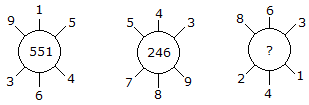A. 262 B. 622 C. 631 D. 824

Explanation:

(915 - 364) = 551

(789 - 543) = 246

(863 - 241) = 622.

37.

Which one will replace the question mark ?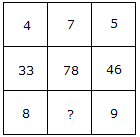A. 12 B. 13 C. 11 D. 10

Explanation:

(4 x 8) + 1 = 33

(5 x 9) + 1 = 46

Similarly, (7 x 11) + 1 = 78.

38.

Which one will replace the question mark ?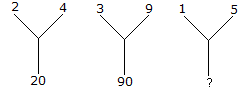A. 20 B. 26 C. 25 D. 75

Explanation:

(2)2 + (4)2 = 20

(3)2 + (9)2 = 90

Therefore, (1)2 + (5)2 = 26.

39.

Which one will replace the question mark ?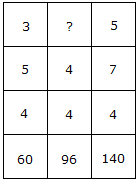A. 4 B. 6 C. 9 D. 8

Explanation:

3 x 5 x 4 = 60

and 5 x 7 x 4 = 140

Therefore, 4 x 4 x ? = 96

? = (96/16) = 6.

40.

Which one will replace the question mark ?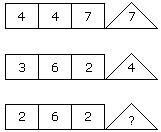A. 2 B. 4 C. 6 D. 8

Explanation:

(4 x 7) % 4 = 7

and (6 x 2) % 3 = 4

Therefore, (6 x 2) % 2 = 6.